Physic Tutorials

How to Solve Questions on Electric Field for Cambridge A level and UTME

How to solve questions on electric field for Cambridge A level and UTME

The answers below give you a step by step on how to solve questions on electric field when writing Cambridge A level physics. Follow the steps and you can comment if you have any question on electric field.

Question 1

Two parallel metal plates, 4.0 cm apart, are at electric potentials of 800 V and 2000 V. Points X, Y and Z are situated in the space between the plates at distances of 1.0 cm, 2.0 cm and 3.0 cm from the lower plate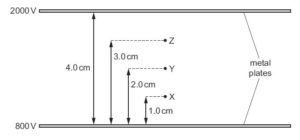What is the electric field strength, in V m–1, at X, Y and Z?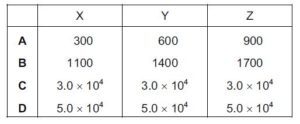{Cambridge A level may/june 2016 p12}

Solution

Electric field strenght at any point in two parallel plate is constant i.e it is a uniform electric field strenght

E = V/d

V is the potential difference between the plate = 2000 – 800 = 1200 v

d is the distance between the plate = 4.0 cm = 0.04 m

E = 1200 / 0.04 = 30000 vm-1 = 3 x 10^4 vm-1

C is the correct answer

You can download physics textbook for free that I have prepared for students writing Cambridge A level, UTME, and ost UTME by Clicking here

Question 2

Two parallel vertical metal plates are connected to a power supply, as shown in Figure below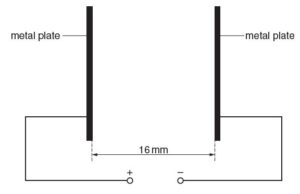An α-particle travels in a vacuum between the two plates. The electric field does work on the α-particle. The gain in kinetic energy of the α-particle is 15 keV. Calculate the electric field strength between the plates

{Cambridge A level 2016 may/june p22}

Solution

workdone = gain in kinetic energy

workdone = force x distance between the plates

workdone = qE x d

The charge on alpha particle = 2e

workdone = qEd = 3.2 x 10^-19 x E x 16 x 10^-3

wordone = 51.2 x 10^-22 E

1eV = 1.6 x 10^-19 J

1KeV = 1.6 x 10 ^-16 J

15 KeV = 24 x 10^-16 J

51.2 x 10^-22 E = 24 x 10^-16 J

E = 24 x 10^-16 / 51.2 x 10^-22

E = 0.46875 x 10 ^6 Vm-1

E = 4.7 x 10^5 Vm-1

Question 3

An oil droplet has charge –q and is situated between two horizontal metal plates as shown in the diagram below.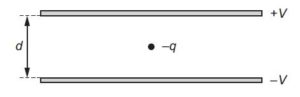The separation of the plates is d. The droplet is observed to be stationary when the upper plate is at potential +V and the lower plate is at potential –V.
For this to occur, what is the weight of the droplet?{Cambridge A level 2015 may/june p11}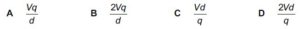Solution

The droplet is stationary because;

the weight on the droplet = the electric force on the droplet

The electric force = qE

E = potential difference / distance between the plate

Potential difference = V – (-V) = 2V

E = 2V / d

Electric force = 2Vq / d

Weight on the droplet = 2Vq/d

B is the correct option

Recommended: Short note on electric field

Bolarinwa Olajire

A lecturer, Educationist, PhD student at FUNAAB, and a Blogger.